•# Fitting equations of state

Ross J. Angel1 and Ian G. Wood2

(1) Virgina Tech Crystallography Laboratory, Virginia Tech, Blacksburg, VA 24060, USA.
E-mail:
rangel@vt.edu ; http://www.crystal.vt.edu/ and (2) Dept. Earth Sciences, University College London, Gower St., London, WC1E 6BT, England.

## Introduction

The rapid developments in high-pressure diffraction techniques over the past decade have made them available as a routine tool to many researchers. To the newcomer in high-pressure research, the topic of Equations of State (EoS), or the relationship between pressure and volume of a solid, appears arcane and complicated by many differing formulations, many errors of derivation in the literature and, to a crystallographer’s view, the frequent use of statistically-dubious fitting procedures. In this brief introduction we outline the issues involved in determining the parameters of isothermal EoS from P-V data and describe the essential elements of a freely available software package, EosFit v5.2 that implements the necessary procedures. Anderson (1995) provides an in-depth analysis of EoS theory, and Fei (1995) outlines the ways in which isothermal EoS can be expanded to describe P-V-T data. The issues involved in fitting EoS are covered in much more detail in Angel (2001), and in the manual accompanying the EosFit v5.2 code, available from http://www.crystal.vt.edu/crystal/software/.

## EoS formulations

There is no fundamental thermodynamically correct formulation for the EoS of a solid, so many have been developed based upon various assumptions about the behaviour of dense solids. There is no reason to suppose any are correct for a given solid, and this must always be remembered when one is attempting to determine EoS parameters through the fitting of P-V or P-V-T data. The most commonly used formulations for EoS are the Birch-Murnaghan, the Vinet and the natural strain (see reviews by Anderson, 1995 and Angel, 2001). The Murnaghan EoS is popular because of its simple functional form, but it does not accurately describe the compression of solids beyond about 10% and should not therefore be used beyond this regime. The issues that arise in fitting EoS can be illustrated by just one of these, the Birch-Murnaghan EoS (Birch, 1947):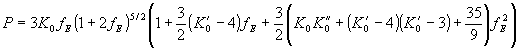in which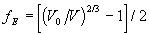. The parameters of the EoS are therefore the bulk modulus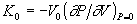and its pressure derivatives,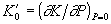and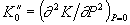, all evaluated at zero pressure, and the zero-pressure volume, V0. This equation is based upon a Taylor expansion of the free energy in terms of fE which can be truncated at any power of fE. Such truncation implies non-zero values for some of the higher-order parameters. Thus, if this EoS is truncated at second-order in the energy then the coefficient of fE must be identical to zero, which requires that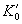has the fixed value of 4 (higher-order terms are ignored). The third-order truncation, in which the coefficient of fE2 is set to zero yields a three-parameter EoS (with V0, K0 and) with an implied value of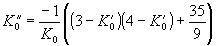. Other EoS yield different implied values for the parameters upon truncation (Anderson, 1995).

Thus a least-squares fit of P-V data to determine the EoS parameters of a solid must proceed in a step-wise fashion:

1. Refine V0and K0 (which scale the entire equation of state) withfixed to the implied value of 4.
2. Refine V0 , K0 and, and test whether the quality of fit as measured by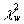has improved and whetherdeviates significantly from the implied value. If it does not, then refinement should be terminated after step 1.
3. If the value ofwas significantly different from the implied value, expand to the next parameter,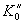, and determine whether it deviates from its implied value. If it does not, then refinement should be terminated after step 2.

These assessments are complicated by the high correlation (often >90%) between the EoS parameters in the least-squares process. This leads some authors into the temptation to fix some parameters (such as V0) while refining higher-order terms thereby biasing the results to the authors’ pre-conceived misconceptions. They forget that V0 is the zero-pressure volume and is not measurable. The room pressure volume is only a close, but biased, estimate of V0. In addition it is not absolutely known but has an associated experimental uncertainty that must be included in a least-squares refinement procedure. This fixing of V0 is often hidden in an fE-FE fit in which the EoS is re-written in terms of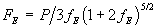which then reduces it to a simple polynomial in fE. But the calculation of both fE and F requires knowledge of V0, so this procedure should never be used to determine EoS parameters. The only statistically valid approach is to refine the EoS parameters directly to a properly weighted dataset.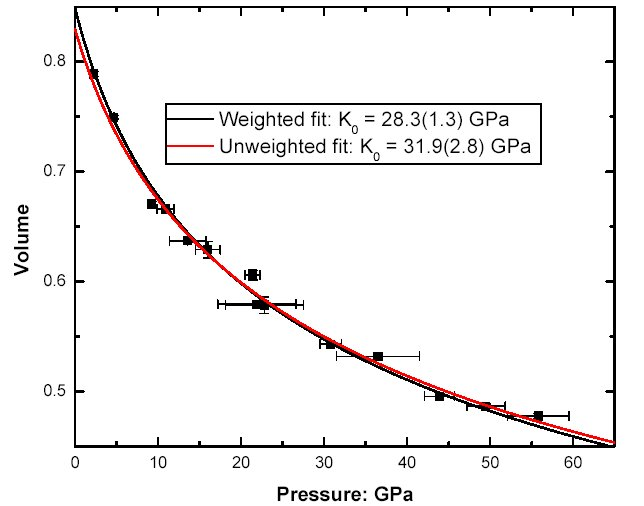Fig 1: An example of the effect of weighting data in a fit of the EoS. Pressure-volume data for the B2 phase of KCl from Campbell and Heinz (1991). The black line is a weighted fit to the data that yields a larger V0 and a smaller K0 than the unweighted fit shown by the red line.

## Implementation

Least-squares fitting of EoS is implemented as part of the EosFit v5.2 program package (Angel, 2001). The least-squares part of the program is derived from an original program written in Basic by Mike Glazer in the late 1970’s and later converted to Fortran by Jenny Piper and Ian Wood. The attraction of this code was that it was written to use external subroutines to define both the value of the function and the necessary derivatives. Although extensively modified for use in the EosFit program, the structure of the original code is retained.

The form of most EoS means that it is simpler to consider volume (and temperature if necessary) as the independent variable in EosFit, with pressure as the dependent variable. The function minimised by least-squares is therefore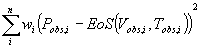in which EoS(V,T) is the pressure calculated for a given V and T from the current set of EoS parameters. Because experimental determinations of EoS data invariably include uncertainties in the volume and temperature measurements the weight assigned to each data point in the least-squares is calculated by the effective variance method (Orear 1982) from all of the available experimental uncertainty estimates: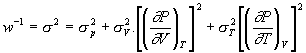There are a number of technical points involved in the derivation of this relationship that may affect the estimates of the values of both the values of the EoS parameters and their uncertainties obtained by least-squares. First, it is assumed that the partial derivatives are constant over the pressure interval between P and P+ δP. This is reasonable provided δP is small compared to the bulk modulus of the material. Second, although the values of σ are correct, the derivatives are incorrectly calculated at the experimentally observed values of V and T rather than at the values estimated by the least-squares fit of the EoS. This leads to a slight over-estimate of the parameter uncertainties (Lybanon 1984, Reed 1992), although the effect is usually insignificant for a slowly varying function such as an EoS with small experimental uncertainties in the data. It is certainly preferable to using unit weights or ignoring the experimental uncertainties in the independent variable, volume. Through the use of thermodynamic identities (e.g. Anderson 1995) the relationship reduces to: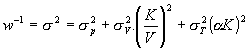. The weights are dependent upon the values of the EoS parameters, and are therefore updated every least-squares cycle.

In EoSFit derivatives are calculated analytically from the current values of the EoS parameters because these appear to lead to more stable least-squares behaviour than derivatives calculated numerically. The normal equations matrix is then solved by the Choleski method. All variables are declared real*8 to avoid round-off errors. Each cycle of least-squares is concluded with the recalculation of the implied values in the EoS. Least-squares refinement is terminated when the sum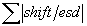for the refined parameters is less than 0.00001. If the value offrom the refinement is greater than unity the variance-covariance matrix is multiplied byprior to the extraction and reporting of the parameter esd’s. As noted above, the correlation between the refined parameters of EoS is quite severe and, in comparing parameter values with other determinations, the covariance must always be considered. EosFit provides the entire variance-covariance matrix, and it is intended to add the calculation of confidence ellipses when time permits.

There are a number of subsidiary programs built into EosFit v5.2 to perform calculations related to EoS. These include calculating volume given the EoS parameters and a pressure and temperature. With the exception of the Murnaghan EoS, the equations relating P, T and V cannot be easily inverted to give V as a function of T and P. Therefore such calculations are performed by a numerical search in which the volume is adjusted until the predicted pressure calculated analytically from the EoS parameters matches the requested pressure to within 0.001%. This level of precision is a somewhat arbitrary choice but is sufficient to prevent any significant rounding errors.

The variation of individual lattice parameters with pressure and temperature is also handled by EosFit. The program substitutes the cube of the lattice parameter for the volume in the equations for the EoS and calculations then proceed as before. The value of "linear-K0" obtained from fitting the isothermal equation in this way is related to the zero-pressure linear compressibility β0 of the axis by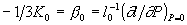in which l0 is the length of the unit-cell axis at zero pressure. Such an approach, while ad-hoc in non-cubic materials, provides values of β0 in good agreement with those derived from experimental measurements of the elastic stiffness tensor.

## References

Anderson OL (1995) Equations of state of solids for geophysics and ceramic science. Oxford University Press, Oxford.

Angel RJ, (2001) Equations of State. In Hazen, R.M., Downs, R.T. (Eds.), High-pressure, high-temperature crystal chemistry. Reviews in Mineralogy and Geochemistry, 41, 35-60.

Birch F (1947) Finite elastic strain of cubic crystals. Phys Rev 71:809-824

Campbell AJ, Heinz DL (1991) Compression of KCl in the B2 structure to 56 GPa. J Phys Chem Solids 52:495-499

Fei Y (1995) Thermal expansion. In: Ahrens TJ (ed) Mineral physics and crystallography, A handbook of physical constants. AGU, Washington DC.

Lybanon M (1984) A better least-squares method when both variables have uncertainties. Am J Phys 52:22-26

Orear J (1982) Least squares when both variables have uncertainties. Am J Phys 50:912-916

Reed BC (1992) Linear least-squares fits with errors in both coordinates. II: Comments on parameter variances. Am J Phys 60:59-62

These pages are maintained by the Commission Last updated: 15 Oct 2021